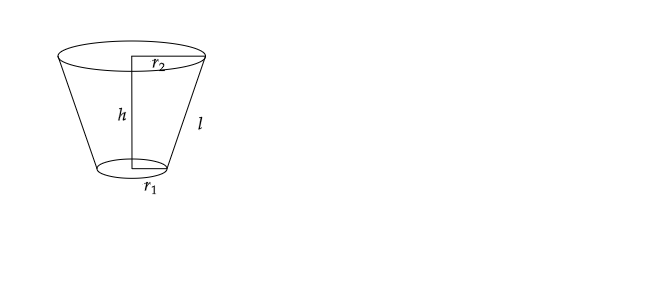# Write the formula for the curved surface area and total surface area of the frustum of a cone.

Frustum of a cone:

When a right circular cone is cut with a plane parallel to the base of the cone, then the solid shape between the plane and the base of the cone is known as the frustum of a cone.

e.g. Bucket is an example of frustum.

Let $r_1=$radius of the larger circular face of the frustum, $r_2=$ radius of the smaller circular face of the frustum, and $l=$ slant height of the frustum.

Curved Surface Area of the frustum of a cone$=\pi ( r_1+r_2)l$.

$l=h^2+( r_1-r_2)^2 and h is the height of the frustum. Total Surface Area of the frustum of a cone$ \pi (r_1+r_2)l+\pi r_{1}^{2}+\pi r_{2}^{2}$Volume of the frustum of a cone$= ( \frac{1}{3}) \pi h( r_{1}^{2} + r_{2}^{2} + r_1 r_2)\$